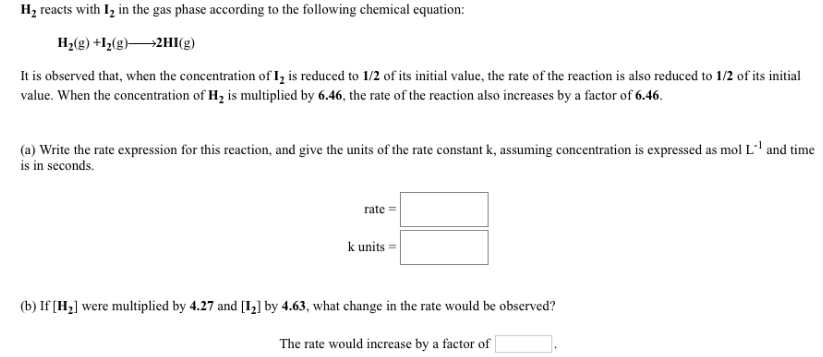# Problem: H2 reacts with I2 in the gas phase according to the following chemical equation: H2 (g) + I2 (g) → 2HI (g) It is observed that, when the concentration of I2 is reduced to 1/2 of its initial value, the rate of the reaction is also reduced to 1/2 of its initial value. When the concentration of H2 is multiplied by 6.46, the rate of the reaction also increases by a factor of 6.46. (a) Write the rate expression for this reaction, and give the units of the rate constant k, assuming concentration is expressed as mol L-1 and time is in seconds. (b) If [H2] were multiplied by 4.27 and [I2] by 4.63, what change in the rate would be observed? The rate would increase by a factor of __________.

###### FREE Expert Solution
82% (121 ratings)###### Problem Details

H2 reacts with I2 in the gas phase according to the following chemical equation:

H2 (g) + I2 (g) → 2HI (g)

It is observed that, when the concentration of I2 is reduced to 1/2 of its initial value, the rate of the reaction is also reduced to 1/2 of its initial value. When the concentration of H2 is multiplied by 6.46, the rate of the reaction also increases by a factor of 6.46.

(a) Write the rate expression for this reaction, and give the units of the rate constant k, assuming concentration is expressed as mol L-1 and time is in seconds.

(b) If [H2] were multiplied by 4.27 and [I2] by 4.63, what change in the rate would be observed?

The rate would increase by a factor of __________.